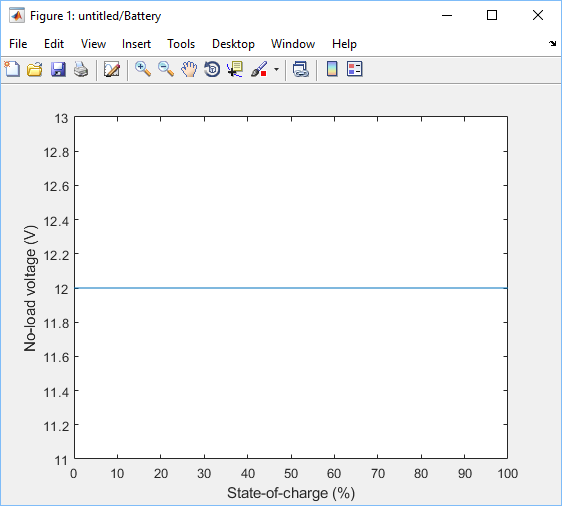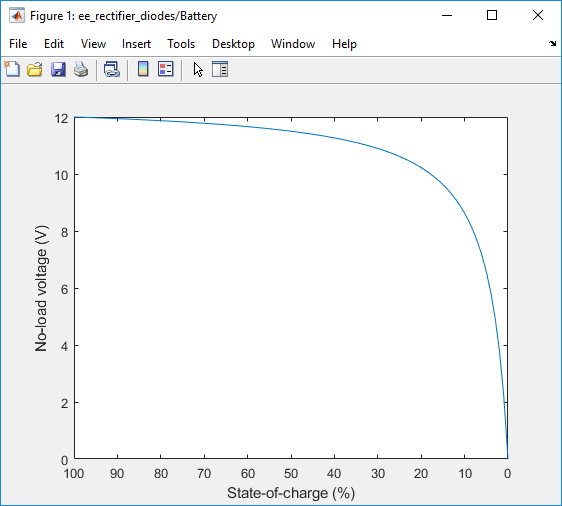## Plot Basic Characteristics for Battery Blocks

You can plot the basic voltage-charge characteristics for the Battery and Battery (Table-Based) blocks based on the current block parameter values without simulating a model.

To plot the basic characteristics, right-click the block and select Electrical > Basic characteristics from the context menu. The software computes a set of bias conditions using the block parameter values and then opens a figure window containing a plot of no-load voltage versus the state of charge (SOC) for the block.

For example, the Basic characteristics option generates this figure for the Battery block with default parameter values.If you change the block parameter values and plot the characteristics again, the plot opens in a new window. You can compare the plots side-by-side to see how the parameter values affect the voltage-charge characteristics. For example, if you set the Battery charge capacity parameter to `Finite` and set the Self-discharge parameter to `Enabled`, and then select the Basic characteristics option again, you generate this plot.